[an error occurred while processing this directive] [an error occurred while processing this directive] [an error occurred while processing this directive] [an error occurred while processing this directive] [an error occurred while processing this directive] [an error occurred while processing this directive] [an error occurred while processing this directive] [an error occurred while processing this directive] [an error occurred while processing this directive] [an error occurred while processing this directive] [an error occurred while processing this directive] /projects/somtoolbox/theory/somalgorithm.shtml [an error occurred while processing this directive] [an error occurred while processing this directive] [an error occurred while processing this directive]The Self-Organizing Map (SOM)

by Teuvo Kohonen

Introduction

The SOM is a new, effective software tool for the visualization of high-dimensional data. It converts complex, nonlinear statistical relationships between high-dimensional data items into simple geometric relationships on a low-dimensional display. As it thereby compresses information while preserving the most important topological and metric relationships of the primary data items on the display, it may also be thought to produce some kind of abstractions. These two aspects, visualization and abstraction, can be utilized in a number of ways in complex tasks such as process analysis, machine perception, control, and communication.

The SOM usually consists of a two-dimensional regular grid of nodes. A model of some observation is associated with each node (cf. Fig. 1).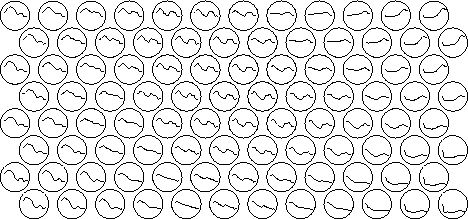Figure 1: In this exemplary application, each processing element in the hexagonal grid holds a model of a short-time spectrum of natural speech (Finnish). Notice that neighboring models are mutually similar.

The SOM algorithm computes the models so that they optimally describe the domain of (discrete or continuously distributed) observations.

The models are organized into a meaningful two-dimensional order in which similar models are closer to each other in the grid than the more dissimilar ones. In this sense the SOM is a similarity graph, and a clustering diagram, too. Its computation is a nonparametric, recursive regression process.

The incremental-learning SOM algorithm

Regression of an ordered set of model vectors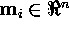into the space of observation vectors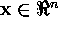is often made by the following process: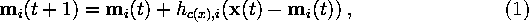where t is the sample index of the regression step, whereby the regression is performed recursively for each presentation of a sample of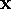. Index c (``winner'') is defined by the condition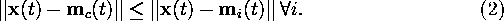Here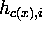is called the neighborhood function, and it is like a smoothing kernel that is time-variable and its location depends on condition in equation (2). It is a decreasing function of the distance between the the ith and cth models on the map grid.

The neighborhood function is often taken to be the Gaussian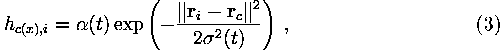where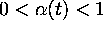is the learning-rate factor, which decreases monotonically with the regression steps,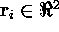and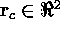are the vectorial locations in the display grid, and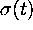corresponds to the width of the neighborhood function, which is also decreasing monotonically with the regression steps.

A simpler definition ofis the following: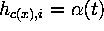if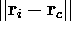is smaller than a given radius around node c (whereby this radius is a monotonically decreasing function of the regression steps, too), but otherwise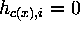. In this case we shall call the set of nodes that lie within the given radius the neighborhood set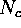.

Due to the many stages in the development of the SOM method and its variations, there is often useless historical ballast in the computations.

For instance, one old ineffective principle is random initialization of the model vectors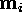. Random initialization was originally used to show that there exists a strong self-organizing tendency in the SOM, so that the order can even emerge when starting from a completely unordered state, but this need not be demonstrated every time. On the contrary, if the initial values for the model vectors are selected as a regular array of vectorial values that lie on the subspace spanned by the eigenvectors corresponding to the two largest principal components of input data, computation of the SOM can be made orders of magnitude faster, since (i) the SOM is then already approximately organized in the beginning, (ii) one can start with a narrower neighborhood function and smaller learning-rate factor.

Many computational aspects like this have been discussed in the software package SOM_PAK , as well as the book .

The batch version of the SOM

Another remark concerns faster algorithms. The incremental regression process defined by equations (1) and (2) can often be replaced by the following batch computation version which, especially with Matlab functions, is significantly faster.

If all observation samples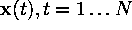are available prior to computations, they can be applied as a batch in the regression, whereby the following computational scheme can be used :

• Initialize the model vectors.
• For each map unit i, collect a list of all those observation samples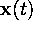, whose most similar model vector belongs to the neighborhood set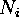of node i.
• Take for each new model vector the mean over the respective list.
• Repeat from step 2 a few times.

Notice that steps 2 and 3 need less memory if at step 2 we only make lists of the observation samplesat those units that have been selected for winner, and at step 3 we form the mean over the union of the lists that belong to the neighborhood setof unit i.

Further remarks

Finally it should be taken into account that the purpose of the SOM is usually visualization of data spaces. For an improved quality (isotropy) of the display it is advisable to select the grid of the SOM units as hexagonal; the reason is similar as when using a hexagonal halftone raster for images.

The above algorithms can often be generalized by defining various generalized matching criteria.

The following categories of similarity graphs, computed by the SOM, have already been used in many practical applications:

• State diagrams for processes and machines
• Data mining applications: similarity graphs for statistical tables and full-text document collections

A list of research papers from very different application areas of the SOM and its variations is available in the Internet at the WWW address http://www.cis.hut.fi/research/som-bibl/.

References

 Kohonen, T., Hynninen, J., Kangas, J., Laaksonen, J. (1996). SOM_PAK: The self-organizing map program package. Report A31. Helsinki University of Technology, Laboratory of Computer and Information Science, Espoo, Finland. Also available in the Internet at the address http://www.cis.hut.fi/research/som_lvq_pak.shtml.

 Kohonen, T. (1995). Self-Organizing Maps. Series in Information Sciences, Vol. 30. Springer, Heidelberg. Second ed. 1997.

You are at: CIS → /projects/somtoolbox/theory/somalgorithm.shtml

Page maintained by webmaster at cis.hut.fi, last updated Friday, 18-Mar-2005 15:53:37 EET

[an error occurred while processing this directive]WWW www.cis.hut.fi